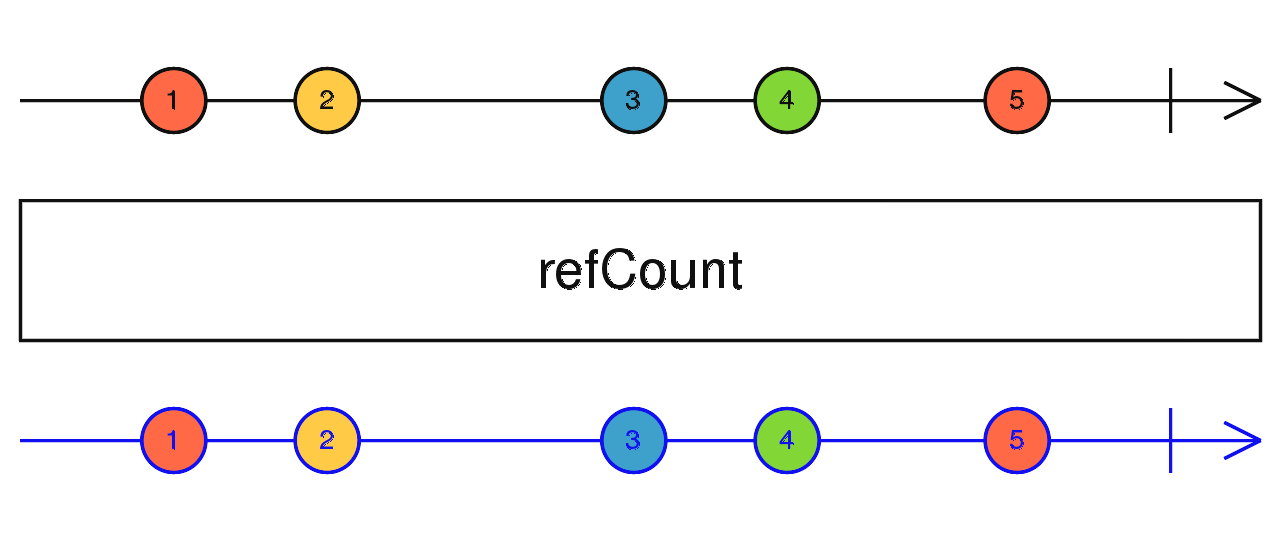/RxJS

# refCount

`function` `stable`

Make a `ConnectableObservable` behave like a ordinary observable and automates the way you can connect to it.

### `refCount<T>(): MonoTypeOperatorFunction<T>`

#### Parameters

There are no parameters.

#### Returns

`MonoTypeOperatorFunction<T>`

## Description

Internally it counts the subscriptions to the observable and subscribes (only once) to the source if the number of subscriptions is larger than 0. If the number of subscriptions is smaller than 1, it unsubscribes from the source. This way you can make sure that everything before the published refCount has only a single subscription independently of the number of subscribers to the target observable.

Note that using the `share` operator is exactly the same as using the publish operator (making the observable hot) and the refCount operator in a sequence.## Example

In the following example there are two intervals turned into connectable observables by using the publish operator. The first one uses the refCount operator, the second one does not use it. You will notice that a connectable observable does nothing until you call its connect function.

```import { interval } from 'rxjs';
import { tap, publish, refCount } from 'rxjs/operators';

// Turn the interval observable into a ConnectableObservable (hot)
const refCountInterval = interval(400).pipe(
tap((num) => console.log(`refCount \${num}`)),
publish(),
refCount()
);

const publishedInterval = interval(400).pipe(
tap((num) => console.log(`publish \${num}`)),
publish()
);

refCountInterval.subscribe();
refCountInterval.subscribe();
// 'refCount 0' -----> 'refCount 1' -----> etc
// All subscriptions will receive the same value and the tap (and
// every other operator) before the publish operator will be executed
// only once per event independently of the number of subscriptions.

publishedInterval.subscribe();
// Nothing happens until you call .connect() on the observable.```